# JEOPARDY Third Nine Weeks 9 weeks review El

• Slides: 27JEOPARDY Third Nine Weeks 9 weeks review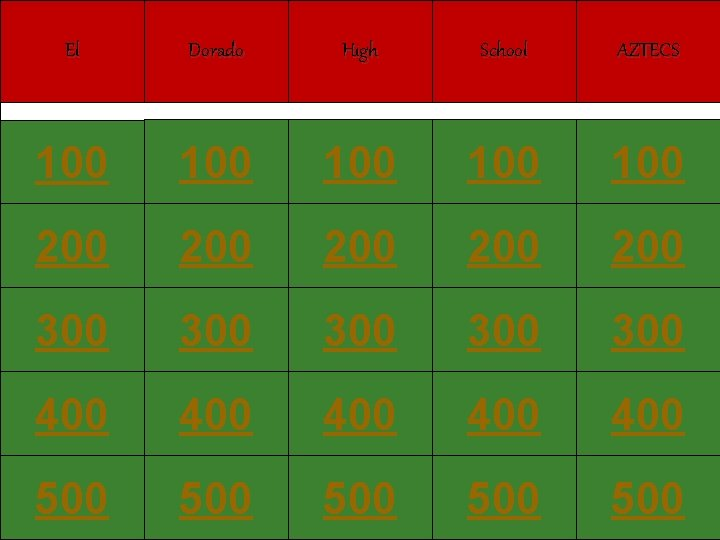El Dorado High School AZTECS 100 100 100 200 200 200 300 300 300 400 400 400 500 500 500Which of the following are found in pure water? A hydroxide ions B hydronium ions C water molecules D all of these A: D. all of these S 2 C 04 Jeopardy ReviewWhat is the concentration of H 3 O+ in pure water at 25 °C? F 10 -7 M G 7. 0 M H 10. 7 M J 107 M A: F. 10 ^-7 S 2 C 04 Jeopardy Review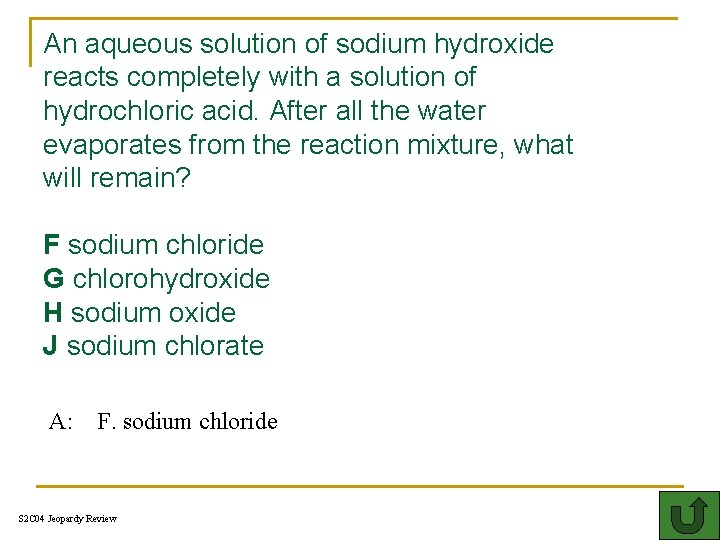An aqueous solution of sodium hydroxide reacts completely with a solution of hydrochloric acid. After all the water evaporates from the reaction mixture, what will remain? F sodium chloride G chlorohydroxide H sodium oxide J sodium chlorate A: F. sodium chloride S 2 C 04 Jeopardy Review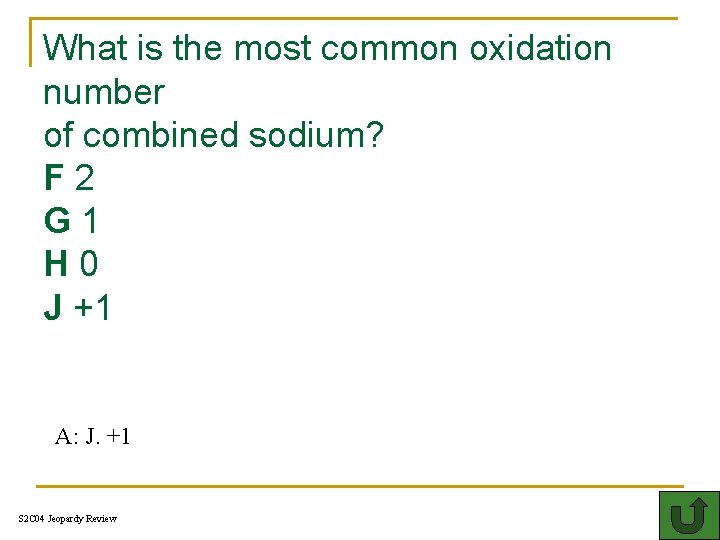What is the most common oxidation number of combined sodium? F 2 G 1 H 0 J +1 A: J. +1 S 2 C 04 Jeopardy ReviewIn balanced chemical equations, the sum of all the oxidation numbers is — A half of the number of elements B always +1 C always – 1 DA: always zero 0 D. always zero S 2 C 04 Jeopardy Review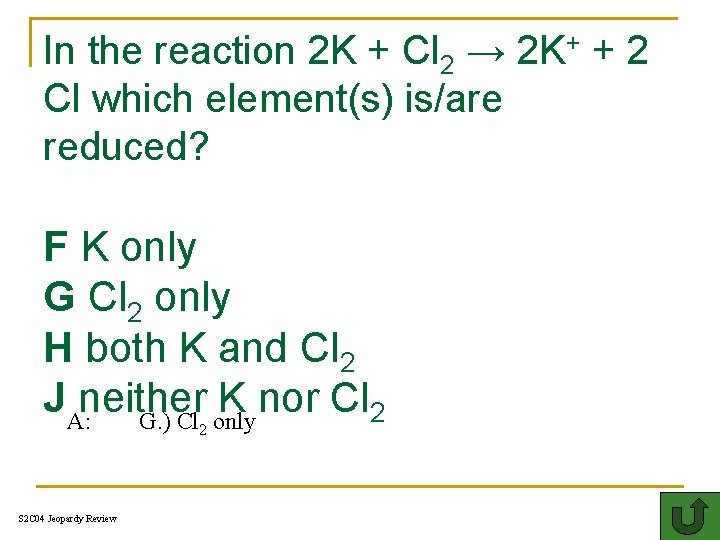In the reaction 2 K + Cl 2 → 2 K+ + 2 Cl which element(s) is/are reduced? F K only G Cl 2 only H both K and Cl 2 JA: neither K nor Cl 2 G. ) Cl only 2 S 2 C 04 Jeopardy Review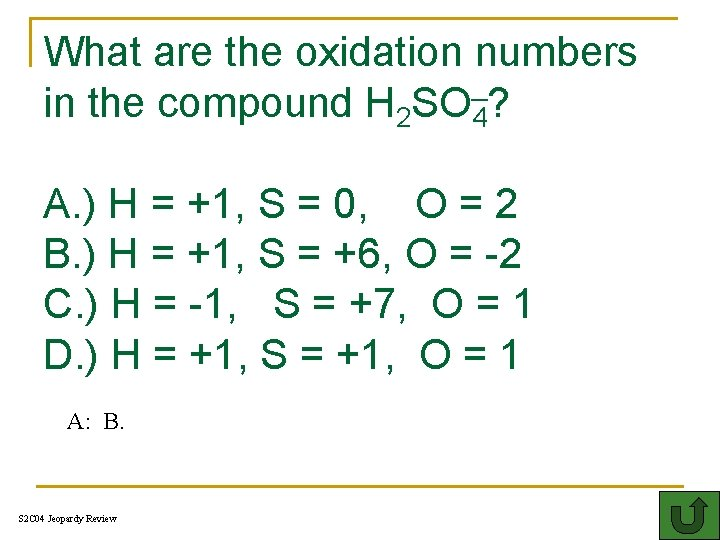What are the oxidation numbers in the compound H 2 SO 4? A. ) H = +1, S = 0, O = 2 B. ) H = +1, S = +6, O = -2 C. ) H = -1, S = +7, O = 1 D. ) H = +1, S = +1, O = 1 A: B. S 2 C 04 Jeopardy Review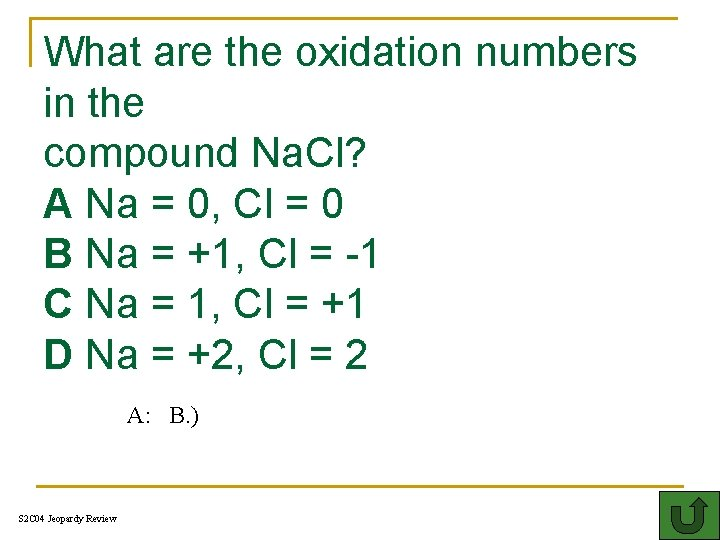What are the oxidation numbers in the compound Na. Cl? A Na = 0, Cl = 0 B Na = +1, Cl = -1 C Na = 1, Cl = +1 D Na = +2, Cl = 2 A: B. ) S 2 C 04 Jeopardy Review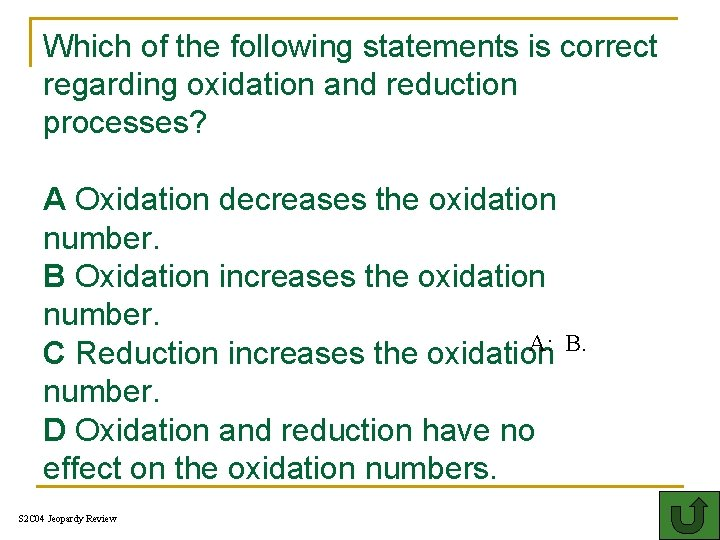Which of the following statements is correct regarding oxidation and reduction processes? A Oxidation decreases the oxidation number. B Oxidation increases the oxidation number. A: B. C Reduction increases the oxidation number. D Oxidation and reduction have no effect on the oxidation numbers. I 400 S 2 C 04 Jeopardy Review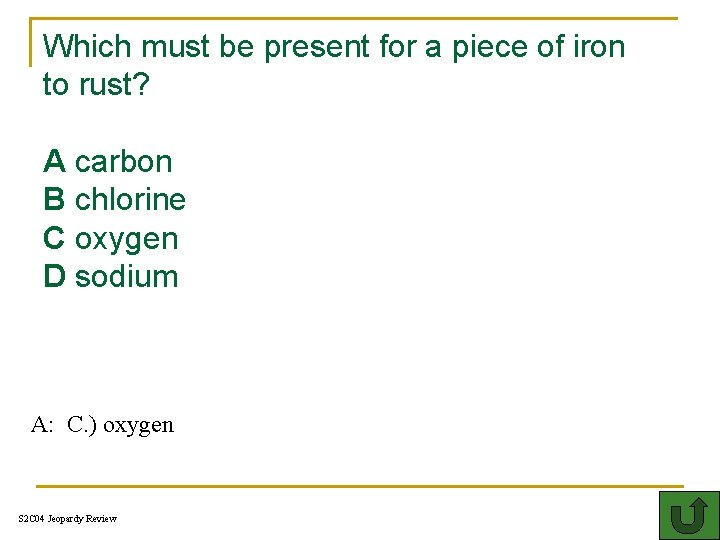Which must be present for a piece of iron to rust? A carbon B chlorine C oxygen D sodium 500 A: C. ) oxygen S 2 C 04 Jeopardy Review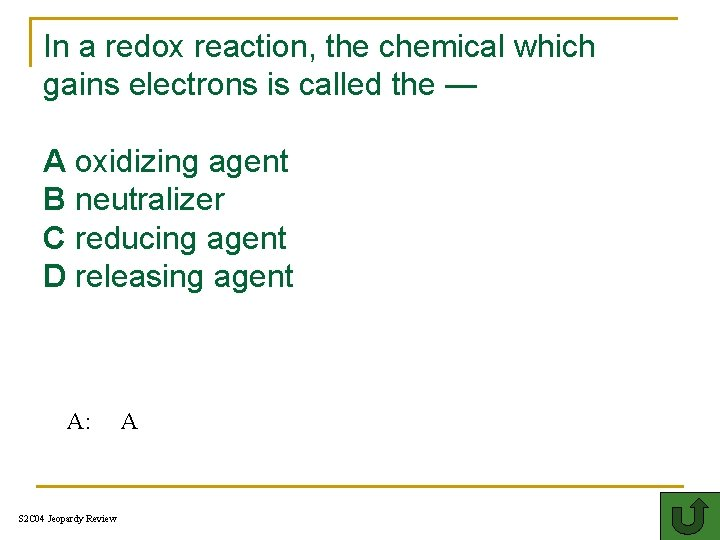In a redox reaction, the chemical which gains electrons is called the — A oxidizing agent B neutralizer TH C reducing agent D releasing agent A: S 2 C 04 Jeopardy Review AWhat is the p. H of a basic solution A: above 7 S 2 C 04 Jeopardy ReviewFor two acids of equal concentration, the stronger acid has — A a higher concentration of hydroxide ions B a higher p. H C a higher concentration of hydronium ions D a stronger bond A: S 2 C 04 Jeopardy Review C.Which of the following is used to calculate the average atomic mass of an element? FH the naturally occurring isotopes G the five most abundant isotopes H the isotopes that are man made J the least common isotopes A: F. the naturally occurring isotopes S 2 C 04 Jeopardy Review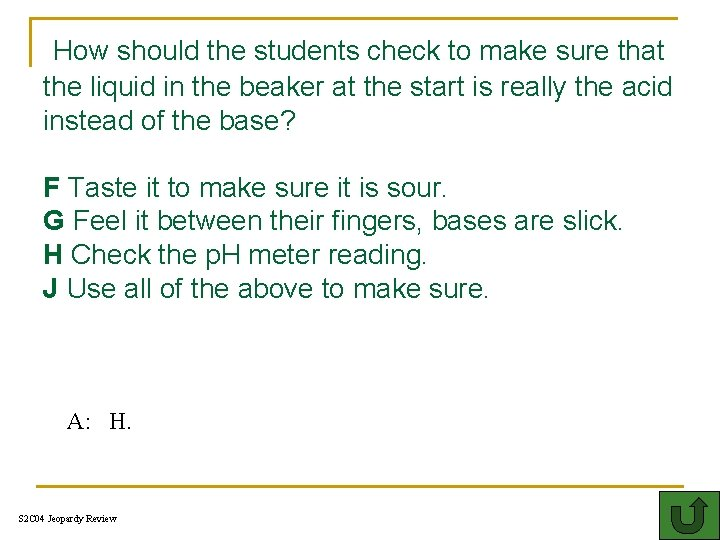How should the students check to make sure that the liquid in the beaker at the start is really the acid instead of the base? F Taste it to make sure it is sour. G Feel it between their fingers, bases are slick. H Check the p. H meter reading. J Use all of the above to make sure. A: H. S 2 C 04 Jeopardy Review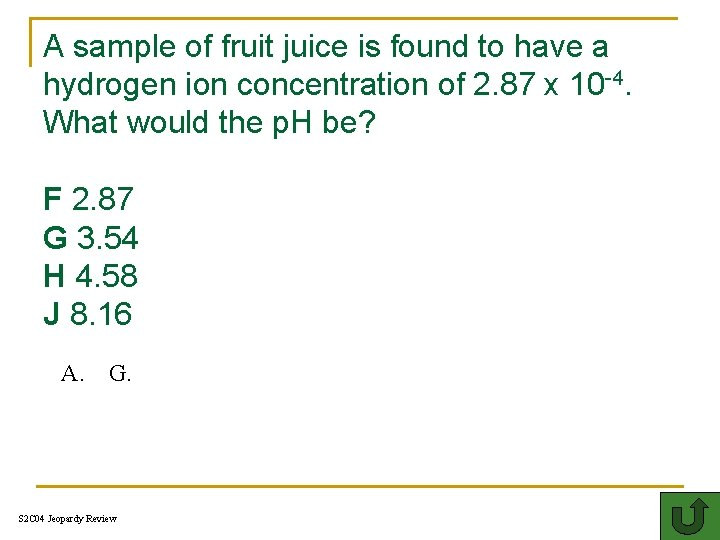A sample of fruit juice is found to have a hydrogen ion concentration of 2. 87 x 10 -4. What would the p. H be? F 2. 87 G 3. 54 H 4. 58 J 8. 16 A. G. S 2 C 04 Jeopardy ReviewWhat is the correct net ionic equation for the following formula: 1 Na 2 CO 3(aq) + 1 Fe. Cl 2(aq) 1 Fe. CO 3(s) + 2 Na. Cl(aq) A. Na+ + Cl- → Na. Cl B. 2 Na+ + 2 Cl- → 2 Na. Cl C. Fe +2 + CO 3 -2 → Fe. CO 3 A + + CO 3 - → Fe. CO 3 D. Fe A. C. S 2 C 04 Jeopardy Review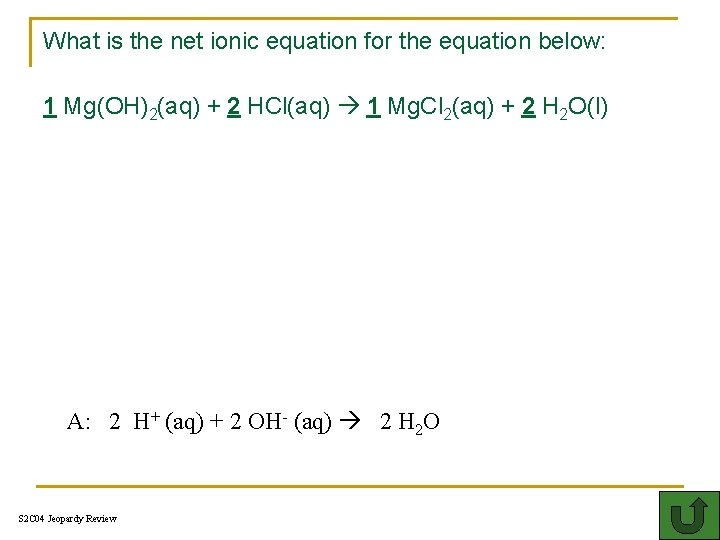What is the net ionic equation for the equation below: 1 Mg(OH)2(aq) + 2 HCl(aq) 1 Mg. Cl 2(aq) + 2 H 2 O(l) A: 2 H+ (aq) + 2 OH- (aq) 2 H 2 O S 2 C 04 Jeopardy ReviewWhat is the p. H of an acidic solution Th A: S 2 C 04 Jeopardy Review below a 7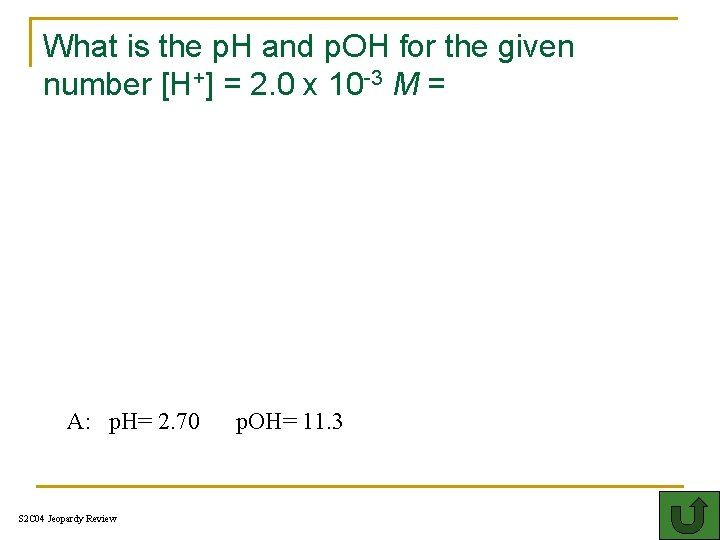What is the p. H and p. OH for the given number [H+] = 2. 0 x 10 -3 M = A: p. H= 2. 70 S 2 C 04 Jeopardy Review p. OH= 11. 3What is the p. H of a solution that has a p. OH of 3. 2? A: p. H= 10. 8 S 2 C 04 Jeopardy ReviewWhat is the p. H of a neutral substance? T A: S 2 C 04 Jeopardy Review 7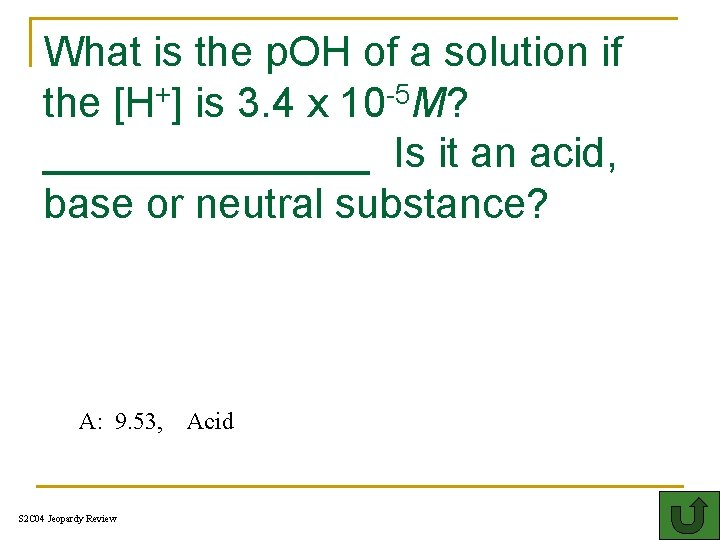What is the p. OH of a solution if the [H+] is 3. 4 x 10 -5 M? _______ Is it an acid, base or neutral substance? T A: 9. 53, S 2 C 04 Jeopardy Review Acid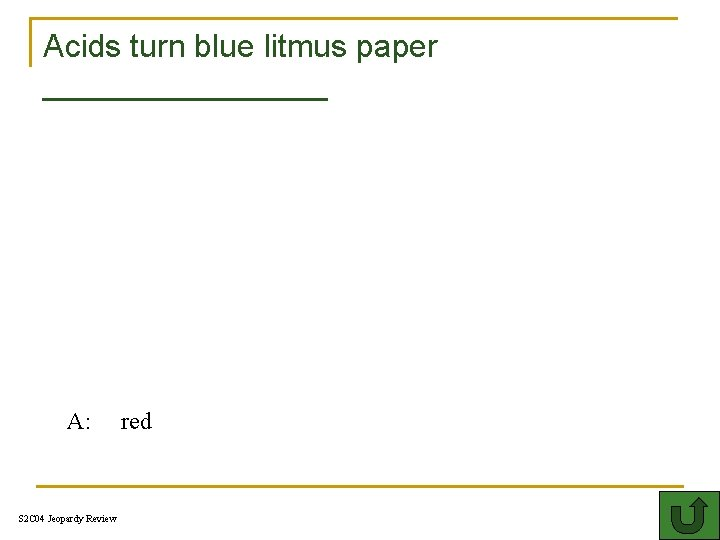Acids turn blue litmus paper ________ T A: S 2 C 04 Jeopardy Review red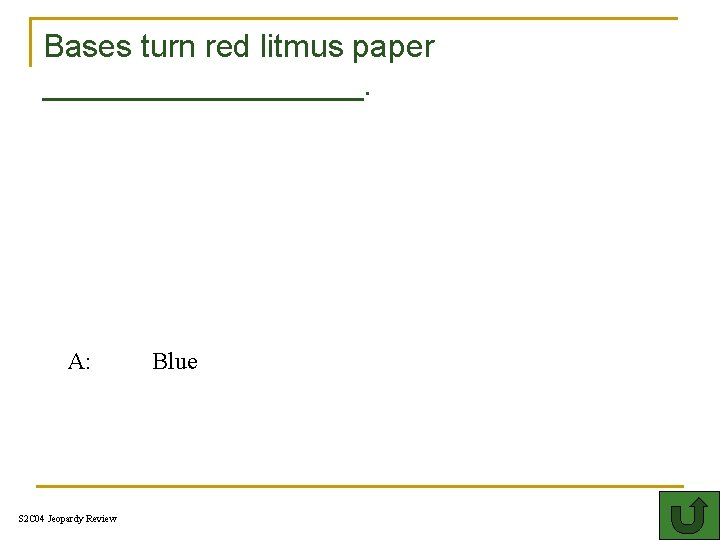Bases turn red litmus paper _________. C A: S 2 C 04 Jeopardy Review Blue